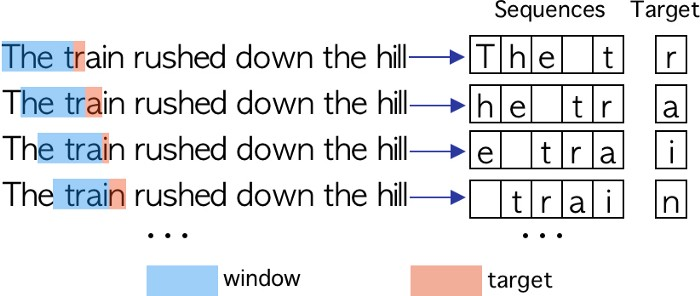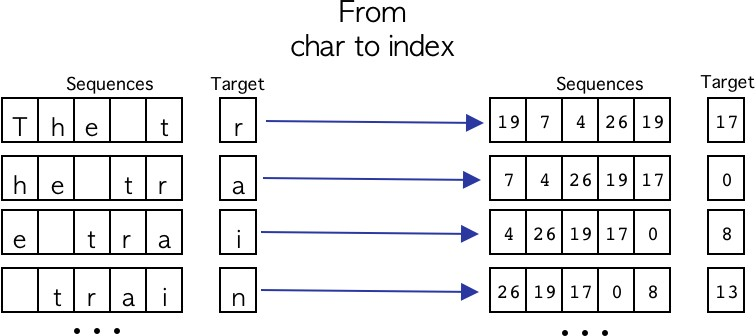# PyTorch:Bi-LSTM的文本生成“写作没有规定。有时它来得容易而且完美；有时就像在岩石上钻孔，然后用炸药把它炸开一样。”—欧内斯特·海明威

• 介绍

• 文本预处理

• 序列生成

• 模型体系结构

• 训练阶段

• 文本生成

### 文本预处理

The train rushed down the hill, with a long shrieking whistle, and then began to go more and more slowly. Thomas had brushed Jack off and thanked him for the coin that he put in his hand, and with the bag in one hand and the stool in the other now went out onto the platform and down the steps, Jack closely following.

def read_dataset(file):
letters = ['a','b','c','d','e','f','g','h','i','j','k','l','m',
'n','o','p','q','r','s','t','u','v','w','x','y','z',' ']

# 打开原始文件
with open(file, 'r') as f:

# 将每一行转换为小写
raw_text = [line.lower() for line in raw_text]

# 创建一个包含整个文本的字符串
text_string = ''
for line in raw_text:
text_string += line.strip()

# 。创建一个字符数组
text = list()
for char in text_string:
text.append(char)

# 去掉所有的符号，只保留字母
text = [char for char in text if char in letters]

return text

text = "The train rushed down the hill."

text = ['t','h','e',' ','t','r','a','i','n',' ','r','u','s','h','e','d',' ','d','o','w','n',
' ','t','h','e',' ','h','i','l','l']

def create_dictionary(text):

char_to_idx = dict()
idx_to_char = dict()

idx = 0
for char in text:
if char not in char_to_idx.keys():

# 构建字典
char_to_idx[char] = idx
idx_to_char[idx] = char
idx += 1

return char_to_idx, idx_to_char

### 序列生成def build_sequences(text, char_to_idx, window):
x = list()
y = list()

for i in range(len(text)):
try:
# 从文本中获取字符窗口
# 将其转换为其idx表示
sequence = text[i:i+window]
sequence = [char_to_idx[char] for char in sequence]

#得到target
# 转换到它的idx表示
target = text[i+window]
target = char_to_idx[target]

# 保存sequence和target
x.append(sequence)
y.append(target)

except:
pass

x = np.array(x)
y = np.array(y)

return x, y

### 模型架构

#### 方法#### Bi-LSTM和LSTMclass TextGenerator(nn.ModuleList):

def __init__(self, args, vocab_size):
super(TextGenerator, self).__init__()

self.batch_size = args.batch_size
self.hidden_dim = args.hidden_dim
self.input_size = vocab_size
self.num_classes = vocab_size
self.sequence_len = args.window

# Dropout
self.dropout = nn.Dropout(0.25)

# Embedding 层

# Bi-LSTM
# 正向和反向
self.lstm_cell_forward = nn.LSTMCell(self.hidden_dim, self.hidden_dim)
self.lstm_cell_backward = nn.LSTMCell(self.hidden_dim, self.hidden_dim)

# LSTM 层
self.lstm_cell = nn.LSTMCell(self.hidden_dim * 2, self.hidden_dim * 2)

# Linear 层
self.linear = nn.Linear(self.hidden_dim * 2, self.num_classes)

# Bi-LSTM
# hs = [batch_size x hidden_size]
# cs = [batch_size x hidden_size]
hs_forward = torch.zeros(x.size(0), self.hidden_dim)
cs_forward = torch.zeros(x.size(0), self.hidden_dim)
hs_backward = torch.zeros(x.size(0), self.hidden_dim)
cs_backward = torch.zeros(x.size(0), self.hidden_dim)

# LSTM
# hs = [batch_size x (hidden_size * 2)]
# cs = [batch_size x (hidden_size * 2)]
hs_lstm = torch.zeros(x.size(0), self.hidden_dim * 2)
cs_lstm = torch.zeros(x.size(0), self.hidden_dim * 2)

# 权重初始化
torch.nn.init.kaiming_normal_(hs_forward)
torch.nn.init.kaiming_normal_(cs_forward)
torch.nn.init.kaiming_normal_(hs_backward)
torch.nn.init.kaiming_normal_(cs_backward)
torch.nn.init.kaiming_normal_(hs_lstm)
torch.nn.init.kaiming_normal_(cs_lstm)

# 从 idx 到 embedding
out = self.embedding(x)

# 为LSTM准备shape
out = out.view(self.sequence_len, x.size(0), -1)

forward = []
backward = []

# 解开Bi-LSTM
# 正向
for i in range(self.sequence_len):
hs_forward, cs_forward = self.lstm_cell_forward(out[i], (hs_forward, cs_forward))
hs_forward = self.dropout(hs_forward)
cs_forward = self.dropout(cs_forward)
forward.append(hs_forward)

# 反向
for i in reversed(range(self.sequence_len)):
hs_backward, cs_backward = self.lstm_cell_backward(out[i], (hs_backward, cs_backward))
hs_backward = self.dropout(hs_backward)
cs_backward = self.dropout(cs_backward)
backward.append(hs_backward)

# LSTM
for fwd, bwd in zip(forward, backward):
input_tensor = torch.cat((fwd, bwd), 1)
hs_lstm, cs_lstm = self.lstm_cell(input_tensor, (hs_lstm, cs_lstm))

# 最后一个隐藏状态通过线性层
out = self.linear(hs_lstm)

batch_size = 64
hidden_size = 128
sequence_len = 100
num_classes = 27

# torch.Size([batch_size, sequence_len])
x : torch.Size([64, 100])

# torch.Size([batch_size, sequence_len, hidden_size])
x_embedded : torch.Size([64, 100, 128])

# torch.Size([sequence_len, batch_size, hidden_size])
x_embedded_reshaped : torch.Size([100, 64, 128])

# hs_forward : torch.Size([batch_size, hidden_size])
hs_forward : torch.Size([64, 128])

# hs_backward : torch.Size([batch_size, hidden_size])
hs_backward: torch.Size([64, 128])

# input_tesor : torch.Size([bathc_size, hidden_size * 2])
input_tensor : torch.Size([64, 256])

# last_hidden_state: torch.Size([batch_size, num_classes])
last_hidden_state: torch.Size([64, 27])

def forward(self, x):

# Bi-LSTM
# hs = [batch_size x hidden_size]
# cs = [batch_size x hidden_size]
hs_forward = torch.zeros(x.size(0), self.hidden_dim)
cs_forward = torch.zeros(x.size(0), self.hidden_dim)
hs_backward = torch.zeros(x.size(0), self.hidden_dim)
cs_backward = torch.zeros(x.size(0), self.hidden_dim)

# LSTM
# hs = [batch_size x (hidden_size * 2)]
# cs = [batch_size x (hidden_size * 2)]
hs_lstm = torch.zeros(x.size(0), self.hidden_dim * 2)
cs_lstm = torch.zeros(x.size(0), self.hidden_dim * 2)

# 权重初始化
torch.nn.init.kaiming_normal_(hs_forward)
torch.nn.init.kaiming_normal_(cs_forward)
torch.nn.init.kaiming_normal_(hs_backward)
torch.nn.init.kaiming_normal_(cs_backward)
torch.nn.init.kaiming_normal_(hs_lstm)
torch.nn.init.kaiming_normal_(cs_lstm)

# 从 idx 到 embedding
out = self.embedding(x)

# 为LSTM准备shape
out = out.view(self.sequence_len, x.size(0), -1)

forward = []
backward = []

# 解开Bi-LSTM
# 正向
for i in range(self.sequence_len):
hs_forward, cs_forward = self.lstm_cell_forward(out[i], (hs_forward, cs_forward))
hs_forward = self.dropout(hs_forward)
cs_forward = self.dropout(cs_forward)
forward.append(hs_forward)

# 反向
for i in reversed(range(self.sequence_len)):
hs_backward, cs_backward = self.lstm_cell_backward(out[i], (hs_backward, cs_backward))
hs_backward = self.dropout(hs_backward)
cs_backward = self.dropout(cs_backward)
backward.append(hs_backward)

# LSTM
for fwd, bwd in zip(forward, backward):
input_tensor = torch.cat((fwd, bwd), 1)
hs_lstm, cs_lstm = self.lstm_cell(input_tensor, (hs_lstm, cs_lstm))

# 最后一个隐藏状态通过线性层
out = self.linear(hs_lstm)

return out

### 训练阶段

def train(self, args):

# 模型初始化
model = TextGenerator(args, self.vocab_size)

# 优化器初始化
optimizer = optim.RMSprop(model.parameters(), lr=self.learning_rate)

# 定义batch数
num_batches = int(len(self.sequences) / self.batch_size)

# 训练模型
model.train()

# 训练阶段
for epoch in range(self.num_epochs):

# Mini batches
for i in range(num_batches):

# Batch 定义
try:
x_batch = self.sequences[i * self.batch_size : (i + 1) * self.batch_size]
y_batch = self.targets[i * self.batch_size : (i + 1) * self.batch_size]
except:
x_batch = self.sequences[i * self.batch_size :]
y_batch = self.targets[i * self.batch_size :]

# 转换 numpy array 为 torch tensors
x = torch.from_numpy(x_batch).type(torch.LongTensor)
y = torch.from_numpy(y_batch).type(torch.LongTensor)

# 输入数据
y_pred = model(x)

# loss计算
loss = F.cross_entropy(y_pred, y.squeeze())

# 清除梯度

# 反向传播
loss.backward()

# 更新参数
optimizer.step()

print("Epoch: %d ,  loss: %.5f " % (epoch, loss.item()))

# 保存权重
torch.save(model.state_dict(), 'weights/textGenerator_model.pt')

### 文本生成

def generator(model, sequences, idx_to_char, n_chars):

# 评估模式
model.eval()

# 定义softmax函数
softmax = nn.Softmax(dim=1)

# 从序列集合中随机选取索引
start = np.random.randint(0, len(sequences)-1)

# 给定随机的idx来定义模式
pattern = sequences[start]

# 利用字典，它输出了Pattern
print("\nPattern: \n")
print(''.join([idx_to_char[value] for value in pattern]), "\"")

# 在full_prediction中，我们将保存完整的预测
full_prediction = pattern.copy()

# 预测开始，它将被预测为一个给定的字符长度
for i in range(n_chars):

# 转换为tensor
pattern = torch.from_numpy(pattern).type(torch.LongTensor)
pattern = pattern.view(1,-1)

# 预测
prediction = model(pattern)
# 将softmax函数应用于预测张量
prediction = softmax(prediction)

# 预测张量被转换成一个numpy数组
prediction = prediction.squeeze().detach().numpy()
# 取概率最大的idx
arg_max = np.argmax(prediction)

# 将当前张量转换为numpy数组
pattern = pattern.squeeze().detach().numpy()
# 窗口向右1个字符
pattern = pattern[1:]
# 新pattern是由“旧”pattern+预测的字符组成的
pattern = np.append(pattern, arg_max)

# 保存完整的预测
full_prediction = np.append(full_prediction, arg_max)

print("Prediction: \n")
print(''.join([idx_to_char[value] for value in full_prediction]), "\"")

window : 100
epochs : 50
hidden_dim : 128
batch_size : 128
learning_rate : 0.001

Seed:
one of the prairie swellswhich gave a little wider view than most of them jack saw quite close to the
Prediction:
one of the prairie swellswhich gave a little wider view than most of them jack saw quite close to the wnd banngessejang boffff we outheaedd we band r hes tller a reacarof t t alethe ngothered uhe th wengaco ack fof ace ca  e s alee bin  cacotee tharss th band fofoutod we we ins sange trre anca y w farer we sewigalfetwher d e  we n s shed pack wngaingh tthe we the we javes t supun f the har man bllle s ng ou   y anghe ond we nd ba a  she t t anthendwe wn me anom ly tceaig t i isesw arawns t d ks wao thalac tharr jad  d anongive where the awe w we he is ma mie cack seat sesant sns t imes hethof riges we he d ooushe he hang out f t thu inong bll llveco we see s the he haa is s igg merin ishe d t san wack owhe o or th we sbe se we we inange t ts wan br seyomanthe harntho thengn  th me ny we ke in acor offff  of wan  s arghe we t angorro the wand be thing a sth t tha alelllll willllsse of s wed w brstougof bage orore he anthesww were ofawe ce qur the he sbaing tthe bytondece nd t llllifsffo acke o t in ir me hedlff scewant pi t bri pi owasem the awh thorathas th we hed ofainginictoplid we me

we, band, pack, the, man, where, he, hang, out, be, thing, me, were

### 结论

#### 参考引用

 LSTM vs. GRU vs. Bidirectional RNN for script generation(https://arxiv.org/pdf/1908.04332.pdf)

 The survey: Text generation models in deep learning(https://www.sciencedirect.com/science/article/pii/S1319157820303360)

http://panchuang.net/

sklearn机器学习中文官方文档：
http://sklearn123.com/

http://docs.panchuang.net/